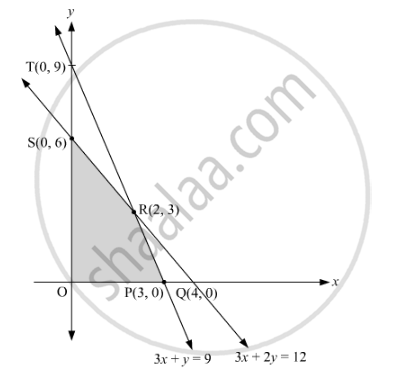# A manufacturer produces two products A and B. Both the products are processed on two differeavailable capacity of first machine is 12 hours and that of second machine is 9 hours per day. - Mathematics and Statistics

Sum

A manufacturer produces two products A and B. Both the products are processed on two different machines. The available capacity of first machine is 12 hours and that of second machine is 9 hours per day. Each unit of product A requires 3 hours on both machines and each unit of product B requires 2 hours on first machine and 1 hour on second machine. Each unit of product A is sold at Rs 7 profit and  B at a profit of Rs 4. Find the production level per day for maximum profit graphically.

#### Solution

Let the numbers of units of products A and B to be produced be x and y, respectively

 Product Machine I (h) II (h) A 3 3 B 2 1

Total profit: Z = 7x + 4y

We have to maximise Z = 7x + 4y, which is subject to constraints.

3x+2y12      (Constraint on machine I)

3x+y 9         (Constraint on machine II)

x0 and y0

The given information can be graphically expressed as follows:Values of Z = 7x + 4y at the corner points are as follows:

 Corner Point Z = 7x + 4y (0, 6) 24 (2, 3) 26 (3, 0) 21

Therefore, the manufacturer has to produce 2 units of product A and 3 units of product B for the maximum profit of Rs 26.

Is there an error in this question or solution?
2015-2016 (March) Delhi Set 1

Share